NCERT Exemplar: Probability

# NCERT Exemplar: Probability - Mathematics (Maths) Class 10

Q.1. In a game, the entry fee is ₹5. The game consists of tossing a coin 3 times. If one or two heads show, Shweta gets her entry fee back. If she throws 3 heads, she receives double the entry fees. Otherwise she will lose. For tossing a coin three times, find the probability that she
(i) loses the entry fee.
(ii) gets double entry fee.
(iii) just gets her entry fee.
Ans.
Possible outcomes when a coin is tossed 3 times:
HHH, HHT, HTH, THH, TTH, THT, HTT, TTT
Total no. of outcomes = 8
(i) Shweta will lose the entry fee if she gets ‘TTT’.
∴ P(Shweta losses the entry fee) = 1/8
(ii) Shweta will get double the entry fee if she gets HHH,
∴ P(Shweta will get double the entry fee) = 1/8.
(iii) Shweta will get her entry fee, if she get HHH,
HTH, THH, TTH, THT or TTT
No. of ways = 6
∴ P(Shweta will get her entry fee) = 6/8 = 3/4.

Q.2. Two dice are thrown at the same time and the product of numbers appearing on them is noted. Find the probability that the product is a prime number.
Ans. Product of the number on the dice is prime number, i.e., 2, 3, 5.
The possible ways are (1, 2), (2, 1), (1, 3), (3, 1), (5, 1), (1, 5).
So, number of possible ways = 6
∴ Required probability = 6/36 = 1/6

Q.3. Apoorv throws two dice once and computes the product of the numbers appearing on the dice. Peehu throws one die and squares the number that appears on it. Who has the better chance of getting the number 36? Why?
Ans. Apoorv throws two dice once.
So, total number of outcomes, n(S) = 36.
Number of outcomes for getting product 36,
n(E1) = 1 [(6 x 6)]
∴ Probability for Apoorv getting the number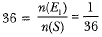Also, Peehu throw one die.
So, total number of outcomes n(S) = 6
Number of outcomes for getting square of a number as 36.
n(E2) = 1   (∵ 62 = 36)
∴ Probability for Peehu getting the number 36 =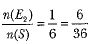Hence, Peehu has better chance of getting the number 36.

Q.4. At a fete, cards bearing numbers 1 to 1000, one number on one card, are put in a box. Each player select one card at random and that card is not replaced. If the selected card has a perfect square greater than 500, the player wins a prize. What is the probability that
(i) the first player wins a prize?
(ii) the second player wins a prize, if the first has won?
Ans.
Number of ways to draw a card by 1st player = 1000
perfect square greater than 500 are 529,576,625,676, 729,784, 841,900, 961
∴ Probability of 1st player winning the prize = 9/1000
when 1st has won the prize then cards left = 999
(because card is not replaced)
Cards with no. which a perfect square greater than 500 = 8(1 winning number removed)
∴ Probability of second player wins a prize when the first player has won = 8/999.

Q.5. There aie 1000 sealed envelopes in a box, 10 of them contain a cash prize of ₹100 each, 100 of them contain a cash prize of ₹ 50 each and 200 of them contain a cash prize of ₹10 each and rest do not contain any cash prize. If they are well shuffled and an envelope is picked up out, what is the probability that it contains no cash prize?
Ans.
Total number of enevelopes = 1000
Let A = envelope contains no cash
Number of envelopes containing no cash = 1000 - (10 + 100 + 200) = 690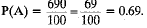Q.6. A bag contains slips numbered from 1 to 100. If Fatima chooses a slip at random from the bag, it will either be an odd number or an even number. Since this situation has only two possible outcomes, so, the probability of each is 1/2.
Ans.
Yes, P (even number) = 50/100 = 1/2;
P (odd number) = 50/100 = 1/2

Q.7. In a family having three children, there may be no girl, one girl, two girls or three girls. So, the probability of each 1/4. Is this correct?
Ans.
Total cases are 8. (GGG), (GGB), (GBG), (GBB), (BGG), (BGB), (BGG), (BBB)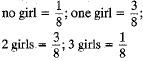Q.8. A student says that if you throw a die, it will show up 1 or not 1. Therefore, the probability of getting 1 and the probability of getting ‘not 1 ’ each is equal to 1/2. Is this correct? Give reasons.
Ans.
No, ∴ P(1) = 1/6
But P(not 1)
It means P(2, 3, 4, 5, 6) = 5/6

Q.9. A die has its six faces marked 0, 1, 1, 1, 6, 6, Two such dice are thrown together and the total score is recorded.
(i) How many different scores are possible?
(ii) What is the probability of getting a total of 7?
Ans.
Possible outcomes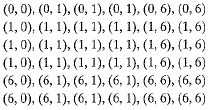Different total scores are 0,1, 6, 2, 7 or 12
Let A = getting a total of 7
No. of favourable outcomes are = 12
∴ P(A) = 12/36 = 1/3

Q.10. A lot consists of 48 mobiles phones of which 42 are good, 3 have only minor defects and 3 have major defects. Vamika will buy a phone if it is good but the trader will only buy a mobile if it has no major defect. One phone is selected at random from the lot. What is the probability that it is
(i) acceptable to Vamika?
Ans.
Total number of phones = 48
Let A = phone is good
Number of good phones = 42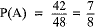∴ Probability that Varnika will buy a phone = 7/8
Let B = Phone has no major defect number of Phones having no major defects = 48 - 3  = 45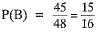∴ Probability that phone is acceptable to the trader = 15/16

The document NCERT Exemplar: Probability | Mathematics (Maths) Class 10 is a part of the Class 10 Course Mathematics (Maths) Class 10.
All you need of Class 10 at this link: Class 10

## Mathematics (Maths) Class 10

115 videos|478 docs|129 tests

## FAQs on NCERT Exemplar: Probability - Mathematics (Maths) Class 10

 1. What is the definition of probability in the context of class 10 mathematics?Ans. In class 10 mathematics, probability is the measure of the likelihood that an event will occur. It is represented as a number between 0 and 1, where 0 indicates impossibility and 1 indicates certainty.
 2. How is probability calculated in class 10 mathematics?Ans. In class 10 mathematics, the probability of an event is calculated by dividing the number of favorable outcomes by the total number of possible outcomes. This gives a ratio or a fraction that represents the likelihood of the event occurring.
 3. What are the different types of events considered in probability for class 10 students?Ans. In probability, class 10 students usually deal with three types of events: sure events, impossible events, and uncertain events. A sure event has a probability of 1, an impossible event has a probability of 0, and uncertain events have probabilities between 0 and 1.
 4. How can we determine the probability of two independent events occurring?Ans. To determine the probability of two independent events occurring, we multiply the probabilities of each event individually. This is known as the multiplication rule of probability. For example, if the probability of event A is 0.4 and the probability of event B is 0.6, the probability of both events occurring is 0.4 * 0.6 = 0.24.
 5. Can the probability of an event exceed 1?Ans. No, the probability of an event cannot exceed 1. In probability theory, the probability of an event is always between 0 and 1, inclusive. A probability of 0 means the event is impossible, while a probability of 1 means the event is certain to occur.

## Mathematics (Maths) Class 10

115 videos|478 docs|129 testsExplore Courses for Class 10 examSignup to see your scores go up within 7 days! Learn & Practice with 1000+ FREE Notes, Videos & Tests.
10M+ students study on EduRev
Track your progress, build streaks, highlight & save important lessons and more!
Related Searches

,

,

,

,

,

,

,

,

,

,

,

,

,

,

,

,

,

,

,

,

,

;